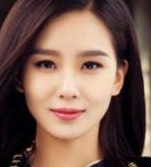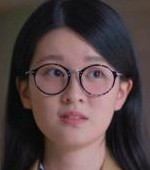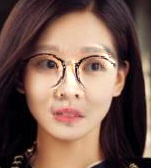# 人工智能几行代码实现换脸，python+dlib实现图文教程# !/usr/bin/python
# -*- coding: utf-8 -*-
# Copyright (c) 2015 Matthew Earl
#
# Permission is hereby granted, free of charge, to any person obtaining a copy
# of this software and associated documentation files (the "Software"), to deal
# in the Software without restriction, including without limitation the rights
# to use, copy, modify, merge, publish, distribute, sublicense, and/or sell
# copies of the Software, and to permit persons to whom the Software is
# furnished to do so, subject to the following conditions:
#
#     The above copyright notice and this permission notice shall be included
#     in all copies or substantial portions of the Software.
#
#     THE SOFTWARE IS PROVIDED "AS IS", WITHOUT WARRANTY OF ANY KIND, EXPRESS
#     OR IMPLIED, INCLUDING BUT NOT LIMITED TO THE WARRANTIES OF
#     MERCHANTABILITY, FITNESS FOR A PARTICULAR PURPOSE AND NONINFRINGEMENT. IN
#     NO EVENT SHALL THE AUTHORS OR COPYRIGHT HOLDERS BE LIABLE FOR ANY CLAIM,
#     DAMAGES OR OTHER LIABILITY, WHETHER IN AN ACTION OF CONTRACT, TORT OR
#     OTHERWISE, ARISING FROM, OUT OF OR IN CONNECTION WITH THE SOFTWARE OR THE
#     USE OR OTHER DEALINGS IN THE SOFTWARE.

"""
This is the code behind the Switching Eds blog post:
http://matthewearl.github.io/2015/07/28/switching-eds-with-python/
See the above for an explanation of the code below.
To run the script you'll need to install dlib (http://dlib.net) including its
Python bindings, and OpenCV. You'll also need to obtain the trained model from
sourceforge:
http://sourceforge.net/projects/dclib/files/dlib/v18.10/shape_predictor_68_face_landmarks.dat.bz2
Unzip with bunzip2 and change PREDICTOR_PATH to refer to this file. The
script is run like so:
If successful, a file output.jpg will be produced with the facial features
from <head image> replaced with the facial features from <face image>.
"""

import cv2
import dlib
import numpy

import sys
# PREDICTOR_PATH = "/home/matt/dlib-18.16/shape_predictor_68_face_landmarks.dat"
PREDICTOR_PATH = "./dat/shape_predictor_68_face_landmarks.dat"
SCALE_FACTOR = 1
FEATHER_AMOUNT = 11

FACE_POINTS = list(range(17, 68))
MOUTH_POINTS = list(range(48, 61))
RIGHT_BROW_POINTS = list(range(17, 22))
LEFT_BROW_POINTS = list(range(22, 27))
RIGHT_EYE_POINTS = list(range(36, 42))
LEFT_EYE_POINTS = list(range(42, 48))
NOSE_POINTS = list(range(27, 35))
JAW_POINTS = list(range(0, 17))

# Points used to line up the images.
ALIGN_POINTS = (LEFT_BROW_POINTS + RIGHT_EYE_POINTS + LEFT_EYE_POINTS +
RIGHT_BROW_POINTS + NOSE_POINTS + MOUTH_POINTS)

# Points from the second image to overlay on the first. The convex hull of each
# element will be overlaid.
OVERLAY_POINTS = [
LEFT_EYE_POINTS + RIGHT_EYE_POINTS + LEFT_BROW_POINTS + RIGHT_BROW_POINTS,
NOSE_POINTS + MOUTH_POINTS,
]

# Amount of blur to use during colour correction, as a fraction of the
# pupillary distance.
COLOUR_CORRECT_BLUR_FRAC = 0.6

detector = dlib.get_frontal_face_detector()
predictor = dlib.shape_predictor(PREDICTOR_PATH)

class TooManyFaces(Exception):
pass

class NoFaces(Exception):
pass

def get_landmarks(im):
rects = detector(im, 1)

if len(rects) > 1:
raise TooManyFaces
if len(rects) == 0:
raise NoFaces

return numpy.matrix([[p.x, p.y] for p in predictor(im, rects).parts()])

def annotate_landmarks(im, landmarks):
im = im.copy()
for idx, point in enumerate(landmarks):
pos = (point[0, 0], point[0, 1])
cv2.putText(im, str(idx), pos,
fontFace=cv2.FONT_HERSHEY_SCRIPT_SIMPLEX,
fontScale=0.4,
color=(0, 0, 255))
cv2.circle(im, pos, 3, color=(0, 255, 255))
return im

def draw_convex_hull(im, points, color):
points = cv2.convexHull(points)
cv2.fillConvexPoly(im, points, color=color)

im = numpy.zeros(im.shape[:2], dtype=numpy.float64)

for group in OVERLAY_POINTS:
draw_convex_hull(im,
landmarks[group],
color=1)

im = numpy.array([im, im, im]).transpose((1, 2, 0))

im = (cv2.GaussianBlur(im, (FEATHER_AMOUNT, FEATHER_AMOUNT), 0) > 0) * 1.0
im = cv2.GaussianBlur(im, (FEATHER_AMOUNT, FEATHER_AMOUNT), 0)

return im

def transformation_from_points(points1, points2):
"""
Return an affine transformation [s * R | T] such that:
sum ||s*R*p1,i + T - p2,i||^2
is minimized.
"""
# Solve the procrustes problem by subtracting centroids, scaling by the
# standard deviation, and then using the SVD to calculate the rotation. See
# the following for more details:
#   https://en.wikipedia.org/wiki/Orthogonal_Procrustes_problem

points1 = points1.astype(numpy.float64)
points2 = points2.astype(numpy.float64)

c1 = numpy.mean(points1, axis=0)
c2 = numpy.mean(points2, axis=0)
points1 -= c1
points2 -= c2

s1 = numpy.std(points1)
s2 = numpy.std(points2)
points1 /= s1
points2 /= s2

U, S, Vt = numpy.linalg.svd(points1.T * points2)

# The R we seek is in fact the transpose of the one given by U * Vt. This
# is because the above formulation assumes the matrix goes on the right
# (with row vectors) where as our solution requires the matrix to be on the
# left (with column vectors).
R = (U * Vt).T

return numpy.vstack([numpy.hstack(((s2 / s1) * R,
c2.T - (s2 / s1) * R * c1.T)),
numpy.matrix([0., 0., 1.])])

im = cv2.resize(im, (im.shape * SCALE_FACTOR,
im.shape * SCALE_FACTOR))
s = get_landmarks(im)

return im, s

def warp_im(im, M, dshape):
output_im = numpy.zeros(dshape, dtype=im.dtype)
cv2.warpAffine(im,
M[:2],
(dshape, dshape),
dst=output_im,
borderMode=cv2.BORDER_TRANSPARENT,
flags=cv2.WARP_INVERSE_MAP)
return output_im

def correct_colours(im1, im2, landmarks1):
blur_amount = COLOUR_CORRECT_BLUR_FRAC * numpy.linalg.norm(
numpy.mean(landmarks1[LEFT_EYE_POINTS], axis=0) -
numpy.mean(landmarks1[RIGHT_EYE_POINTS], axis=0))
blur_amount = int(blur_amount)
if blur_amount % 2 == 0:
blur_amount += 1
im1_blur = cv2.GaussianBlur(im1, (blur_amount, blur_amount), 0)
im2_blur = cv2.GaussianBlur(im2, (blur_amount, blur_amount), 0)

# Avoid divide-by-zero errors.
im2_blur += (128 * (im2_blur <= 1.0)).astype(im2_blur.dtype)

return (im2.astype(numpy.float64) * im1_blur.astype(numpy.float64) /
im2_blur.astype(numpy.float64))

M = transformation_from_points(landmarks1[ALIGN_POINTS],
landmarks2[ALIGN_POINTS])

axis=0)

warped_im2 = warp_im(im2, M, im1.shape)
warped_corrected_im2 = correct_colours(im1, warped_im2, landmarks1)

cv2.imwrite('output.jpg', output_im)

12-234803

#### 再也不要相信你的眼睛：步步逼近的AI换脸术

09-11147

#### 人工智能几行代码实现换脸07-231037

#### Python, dlib, OpenCV换脸进行时（转）

06-151万+

#### AI换脸技术源码

10-266438

#### 很吓人的技术，200行Python代码实现换脸程序

09-283万+

#### 图像识别的原理、过程、应用前景，精华篇！

05-247054

#### 人工智能python+dlib+opencv技术10分钟实现抖音人脸变狗头详细图文教程和完整项目代码

04-28543

#### python实现一键换脸+源码+教程

04-17469

#### 萌新如何用Python实现人脸替换？

06-145673

#### 两张二维人物图像互相换脸代码

08-045165

#### 教你用200行Python代码“换脸”

04-075万+

#### Synchronized关键字深析（小白慎入，深入jvm源码，两万字长文）

07-058147

#### python利用dlib换脸，亲测可用

04-077万+

#### 超全Python图像处理讲解（多图预警）

01-2130万+

#### Java校招入职华为，半年后我跑路了

12-091万+

#### 视频换脸-Deepfakes代码解读和训练说明

05-074万+

#### Auto.JS实现抖音，刷宝等刷视频app,自动点赞，自动滑屏，自动切换视频

07-1021万+

#### Java 最常见的 200+ 面试题：面试必备©️2020 CSDN 皮肤主题: 大白 设计师: CSDN官方博客点击重新获取扫码支付1.余额是钱包充值的虚拟货币，按照1:1的比例进行支付金额的抵扣。
2.余额无法直接购买下载，可以购买VIP、C币套餐、付费专栏及课程。余额充值